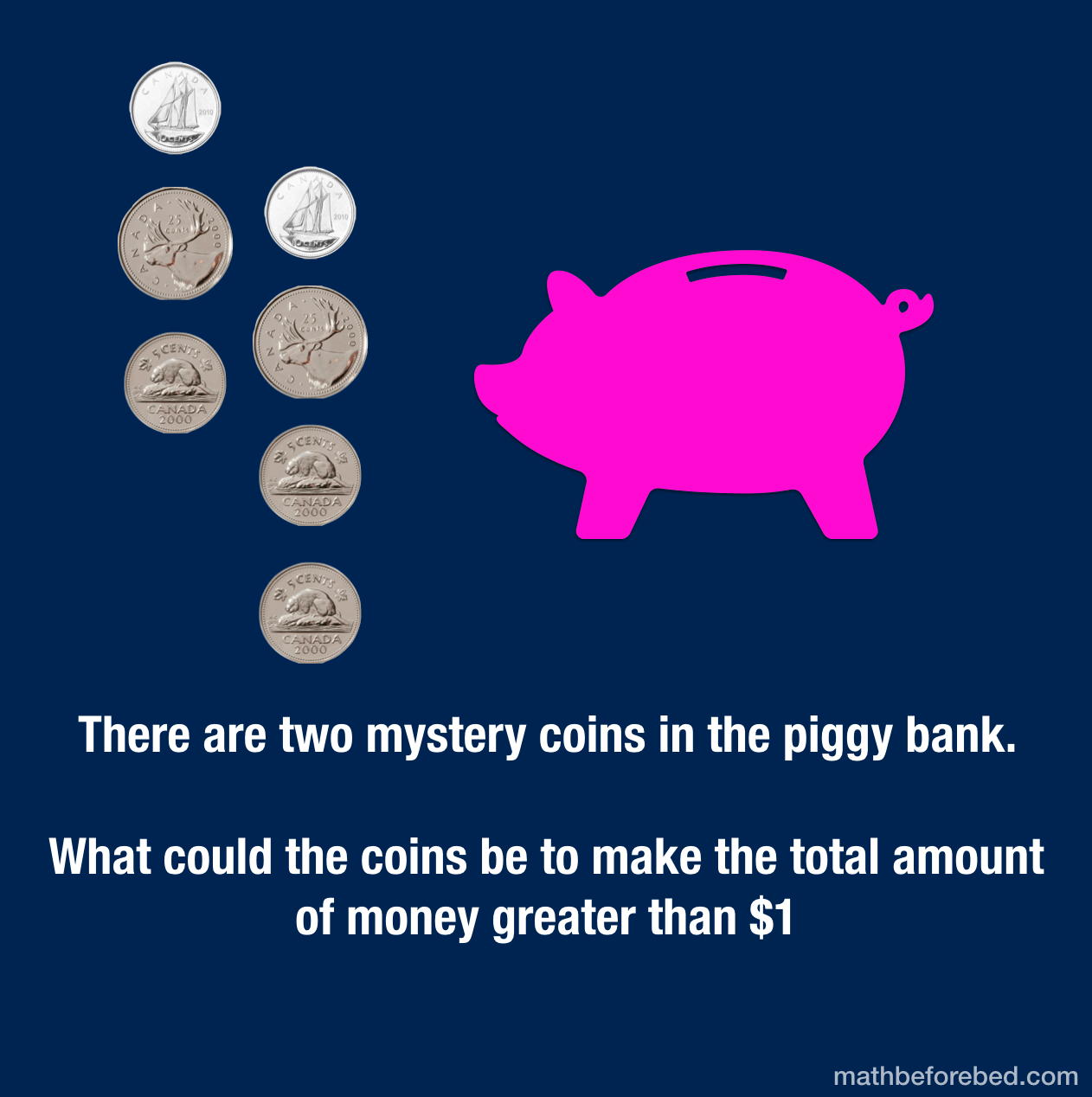# Mystery BankThere are two mystery coins in the piggy bank. What could the coins be to make the total amount of money greater than \$1

## 11 thoughts on “Mystery Bank”

1.ruvel fagura says:

so
10+10+25+25+5+5+5=85

2.YIYI says:

1.35 because
25+25=50
50+10=60
60+10=70
70+5=75
75+5=80
80+5=85
85+25=110
110+25=135

3.sloane says:

.85+.25=1.10
.50+.65=1.15
.70+.80=1.50
.75+.50=1.25
.60+.45=1.05
.80+.50=1.30
.65+.45=1.10
.55+75=1.30

4.sloane says:

.85+.50=1.35
.85+.20=1.05

5.Yuvan says:

Its 85 cents and the other two mystery coins are 10 and 10, making the total to 1.05

6.Yuvan says:

o.85 + 0.10 + 0.10 = 1.05
0.85+ 0.10 + 0.25 = 1.20
0.85 +0.25 +0.25 = 1.35
0.85+0.25+0.05 = 1.15

4 different sums that makes greater than \$1.

7.sloane says:

the mystery coins might be
two .10 cents….85 cents +.10+.10=1.05
.25 and .5………85 cents +.25+.5=1.15
.25 and .10…….85 cents +.25+.10=1.20
two .25 cent….85 cents +.25+.25=1.35

8.Ruhan says:

Ruhan says you first add: 25cents + 25 cents = 50 cents, you then add 10 cents + 10 cents to 50 cents = 70 cents, then you add 5 cents + 5 cents + 5 cents to 70 cents = 85 cents total that we have. The piggy bank can have either a loonie (\$1= 100 cents), or it could have a toonie ( \$2= 200 cents). Then we add \$85 cents + 100 cents = \$1.85, and we can 85 cents + 200 cents = \$2.85 cents. This means the missing coins that are in the piggy’s belly are \$1 and \$2. If you had \$1 or \$2 or 85 cents, you will have MORE than \$1 total amount of money. Ruhan did need help arriving at this, but we broke down the math to show how many cents make up each value of the coin.

9.Kayal says:

10+10=20 25+25=50 10+5+5=20 =60 60 +5=75 +two 25¢ = 125

10.Ashley says:

10+10+25+25+5+5+5=85+10+5=100
100 equals 1 dollar.
So the 2 mystery coins are 10 and 5.

11.Damien says:

If the coins not in the piggy bank is 85 cents the mystery coins are a quarter making 1.35